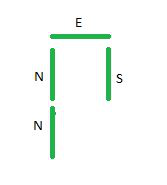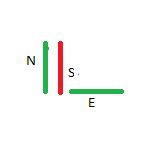Related Articles

# Total time required to travel a path denoted by a given string

• Last Updated : 28 Jun, 2021

Given a string path consisting of characters ‘N’, ‘S’, ‘E’ and ‘W’ denoting 1 unit movement in North, South, East, and West directions respectively, the task is to find the time taken to travel the complete path starting from the origin, if it takes 2 and 1 minutes to travel on an unvisited and visited segment respectively.

Examples :

Input: path = “NNES”
Output : 8Explanation: Since every segment is visited only once, cost = 2 * 4 = 8.

Input : path = “NSE”
Output : 5Explanation:
Step 1: Travel north. Time Taken = 2 minutes.
Step 2: Travel south on that same visited segment. Time Taken = 1 minutes.
Step 3: Travel east.Time Taken = 2 minutes. Therefore, total time taken = 2 + 1 + 2 = 5.

Approach: The idea is to use a Set to store all the visited segments and before visiting each segment, check if it is present in the Set or not. Follow the steps below to solve the problem.

• Initialize a set s to store a pair of integers. The set will store all visited segments.
• Initialize two integers x = 0 and y = 0 denoting the current position. Also, initialize a variable time = 0 to store the total time needed to travel the complete path.
• Traverse the string and follow the below steps
• Initialize two integers p and q to x and y respectively.
• If path[i] is equal to ‘N’ increment y, else if path[i] is equal to ‘S’ decrement y, else if path[i] is equal to ‘E’ increment x, otherwise decrement x.
• Check if the segment {p+x, q+y} exists in the set or not. if it does add 1 to the value of time otherwise add 2 to the value of time.
• Insert the segment {p+x, q+y} into the set.
• After completing the above steps print the value of time.

Below is implementation of the above approach.

## C++

 `// C++ code for the above approach``#include ``using` `namespace` `std;` `// Function to calculate time``// taken to travel the path``void` `calcTotalTime(string path)``{``    ``// Stores total time``    ``int` `time` `= 0;` `    ``// Initial position``    ``int` `x = 0, y = 0;` `    ``// Stores visited segments``    ``set > s;` `    ``for` `(``int` `i = 0; i < path.size(); i++) {` `        ``int` `p = x;``        ``int` `q = y;` `        ``if` `(path[i] == ``'N'``)``            ``y++;` `        ``else` `if` `(path[i] == ``'S'``)``            ``y--;` `        ``else` `if` `(path[i] == ``'E'``)``            ``x++;` `        ``else` `if` `(path[i] == ``'W'``)``            ``x--;` `        ``// Check whether segment``        ``// is present in the set``        ``if` `(s.find({ p + x, q + y })``            ``== s.end()) {``            ``// Increment the value``            ``// of time by 2``            ``time` `+= 2;` `            ``// Insert segment into the set``            ``s.insert({ p + x, q + y });``        ``}``        ``else``            ``time` `+= 1;``    ``}` `    ``// Print the value``    ``// of time``    ``cout << ``time` `<< endl;``}` `// Driver Code``int` `main()``{``    ``string path = ``"NSE"``;` `    ``calcTotalTime(path);` `    ``return` `0;``}`

## Java

 `// Java program for above approach``import` `java.util.*;` `class` `GFG{` `// Function to calculate time``// taken to travel the path``static` `void` `calcTotalTime(String path)``{``    ` `    ``// Stores total time``    ``int` `time = ``0``;` `    ``// Initial position``    ``int` `x = ``0``, y = ``0``;` `    ``// Stores visited segments``    ``Set s = ``new` `HashSet<>();``    ``for``(``int` `i = ``0``; i < path.length(); i++)``    ``{``        ``int` `p = x;``        ``int` `q = y;` `        ``if` `(path.charAt(i) == ``'N'``)``            ``y++;` `        ``else` `if` `(path.charAt(i) == ``'S'``)``            ``y--;` `        ``else` `if` `(path.charAt(i) == ``'E'``)``            ``x++;` `        ``else` `if` `(path.charAt(i) == ``'W'``)``            ``x--;` `        ``// Check whether segment``        ``// is present in the set``        ``String o = (p + x) + ``" "` `+ (q + y);``        ``if` `(!s.contains(o))``        ``{``            ` `            ``// Increment the value``            ``// of time by 2``            ``time += ``2``;` `            ``// Insert segment into the set``            ``s.add(o);``        ``}``        ``else``            ``time += ``1``;``    ``}` `    ``// Print the value``    ``// of time``    ``System.out.println(time);``}` `// Driver Code``public` `static` `void` `main(String[] args)``{``    ``String path = ``"NSE"``;` `    ``calcTotalTime(path);``}``}` `// This code is contributed by Hritik`

## Python3

 `# Python 3 code for the above approach` `# Function to calculate time``# taken to travel the path``def` `calcTotalTime(path):` `    ``# Stores total time``    ``time ``=` `0` `    ``# Initial position``    ``x ``=` `0``    ``y ``=` `0` `    ``# Stores visited segments``    ``s ``=` `set``([])` `    ``for` `i ``in` `range``(``len``(path)):` `        ``p ``=` `x``        ``q ``=` `y` `        ``if` `(path[i] ``=``=` `'N'``):``            ``y ``+``=` `1` `        ``elif` `(path[i] ``=``=` `'S'``):``            ``y ``-``=` `1` `        ``elif` `(path[i] ``=``=` `'E'``):``            ``x ``+``=` `1` `        ``elif` `(path[i] ``=``=` `'W'``):``            ``x ``-``=` `1` `        ``# Check whether segment``        ``# is present in the set``        ``if` `(p ``+` `x, q ``+` `y) ``not` `in` `s:``            ``# Increment the value``            ``# of time by 2``            ``time ``+``=` `2` `            ``# Insert segment into the set``            ``s.add((p ``+` `x, q ``+` `y))` `        ``else``:``            ``time ``+``=` `1` `    ``# Print the value``    ``# of time``    ``print``(time)`  `# Driver Code``if` `__name__ ``=``=` `"__main__"``:` `    ``path ``=` `"NSE"` `    ``calcTotalTime(path)` `    ``# This code is contributed by ukasp.`

## C#

 `// C# program for the above approach``using` `System;``using` `System.Collections.Generic;` `class` `GFG{` `// Function to calculate time``// taken to travel the path``static` `void` `calcTotalTime(``string` `path)``{``    ` `    ``// Stores total time``    ``int` `time = 0;` `    ``// Initial position``    ``int` `x = 0, y = 0;` `    ``// Stores visited segments``    ``HashSet<``string``> s = ``new` `HashSet<``string``>();``    ``for``(``int` `i = 0; i < path.Length; i++)``    ``{``        ``int` `p = x;``        ``int` `q = y;` `        ``if` `(path[i] == ``'N'``)``            ``y++;` `        ``else` `if` `(path[i] == ``'S'``)``            ``y--;` `        ``else` `if` `(path[i] == ``'E'``)``            ``x++;` `        ``else` `if` `(path[i] == ``'W'``)``            ``x--;` `        ``// Check whether segment``        ``// is present in the set``        ``string` `o = (p + x) + ``" "` `+ (q + y);``        ``if` `(s.Contains(o) == ``false``)``        ``{``            ` `            ``// Increment the value``            ``// of time by 2``            ``time += 2;` `            ``// Insert segment into the set``            ``s.Add(o);``        ``}``        ``else``            ``time += 1;``    ``}` `    ``// Print the value``    ``// of time``    ``Console.Write(time);``}` `// Driver Code``public` `static` `void` `Main()``{``    ``string` `path = ``"NSE"``;` `    ``calcTotalTime(path);``}``}` `// This code is contributed by bgangwar59`

## Javascript

 ``
Output:
`5`

Time Complexity: O(NlogN)
Auxiliary Space: O(N)

Attention reader! Don’t stop learning now. Get hold of all the important mathematical concepts for competitive programming with the Essential Maths for CP Course at a student-friendly price. To complete your preparation from learning a language to DS Algo and many more,  please refer Complete Interview Preparation Course.

My Personal Notes arrow_drop_up